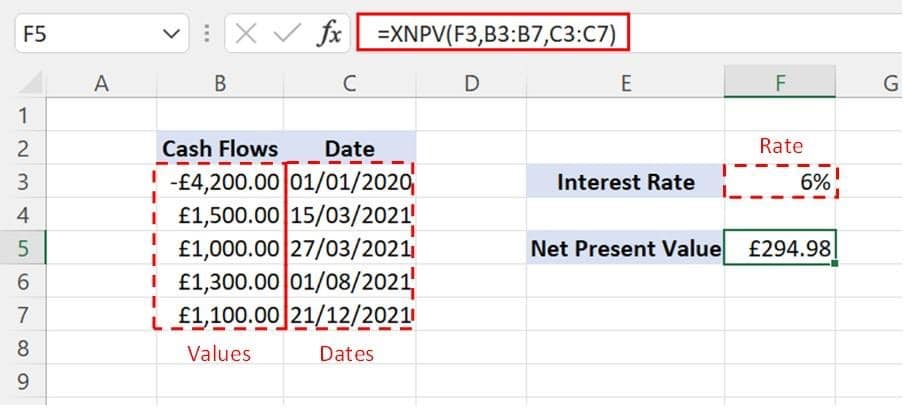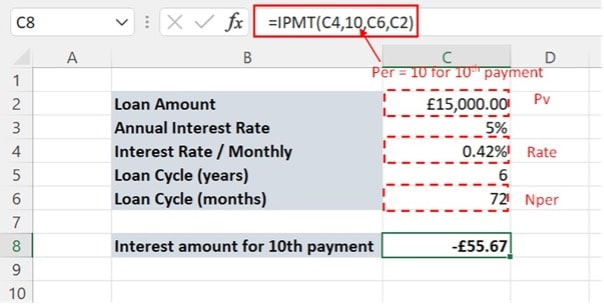Connect with us

# The 5 Financial Excel Functions Every Entrepreneur Must KnowPublished

onEntrepreneurship is full of challenges that you need to overcome to make your idea a success.

A key skill that you need to learn is managing your finances.

Luckily, Microsoft Excel offers a lot of functions and features that you can master in no time.

With the help of a provider of financial modelling training, Acuity Training, let’s look at the top 5 financial Excel functions that every entrepreneur needs to know.

1. ## XNPV

XNPV is used to evaluate the business by calculating the net present value of future cash flows.

It is the improved version of NPV.

The NPV function assumes the duration between cash flows is equal, which can be unrealistic.

The XNPV lets you define the exact date for each cash flow. This way the calculation becomes more precise.

The XNPV function contains three arguments

=XNPV(rate, values, dates)

Rate: Discount rate as a percentage

Values: Actual cash flow values

Dates: Exact date of each cash flow

Let’s understand the working of XNPV with a simple example.

XNPV is used to calculate the net present value for the cashflows in cell B3:B7.

Cells C3:C7 contains the dates for each cashflow and cell F3 contains the rate.Entrepreneurs can use XNPV to calculate the net present values based on the actual outcomes to compare them with the projected cash flows.

1. ## XIRR

XIRR calculates the internal rate of return of a project by considering the cash flows and the actual dates of cash flows.

Many people see it as the XNPV function but operating the other way round as it gives you the interest rate at which the project breaks even, rather than the ‘profit’ at a given rate of return.

The XIRR function has three simple arguments

=XIRR(values, dates, [guess])

Values: Cash flow values

Dates: Actual dates for each cash flow

Guess: [Optional] Expected value of IRR. Set at 0.1 (10%) by default

Below is the example of using XIRR to calculate the internal rate of return.

By assigning cell B3:B7 as Values and cell C3:C7 as Dates, Excel gives us that the IRR is 11%.

Entrepreneurs can use the XIRR function to evaluate the actual returns on a project and compare them with the projected returns to keep things in control.

Similarly, if you reverse the signs, this is a good way of working out the true interest rate on a return if you include all the relevant cashflows.

1. ## MIRR

MIRR is the modified rate of return and is used to calculate the internal rate of return but taking into account your alternative possibilities.

If you have a project that pays you 10%, then it is more valuable to you if your next best opportunity pays you 1% than if your next best option pays you 6%.

This mirrors the real world more closely, where excess funds will be invested in other revenue-generating activities or the bank to earn interest. For fuller details, see this article on the MIRR formula.

The XIRR and IRR (which it replaces) functions do not consider the reinvestment rate when calculating a return rate.

The MIRR formula is simple to use

= MIRR(values, finance_rate, reinvest_rate)

Values: Refers to the series of cashflows

Finance_rate: Interest rate for the borrowed amount

Reinvest_rate: The interest rate that will be generated through reinvestment of cash.

Let’s calculate the modified internal rate for a simple series of cashflows.

By assigning cell B3:B7 as Values, cell E3 as Finance_rate, and cell E5 as Reinvest_rate, our MIRR was calculated as 9%.

MIRR function is helpful for entrepreneurs in calculating the internal rate while considering different options for reinvestment.

1. ## PMT

The PMT function is used to calculate the periodic payments for loans.

PMT function considers three inputs, the borrowed amount, the number of instalments, and the borrowing rate when calculating the payments.

The output calculated by the PMT includes both the principal and interest amount.

PMT assumes that the interest rate will be constant throughout the loan’s lifetime.

=PMT(Rate, Nper, Pv, [Fv], [Type])

Rate: Refers to the borrowing rate

Nper: Total number of repayments

Pv: Present value of the loan amount

Fv: [Optional] Outstanding amount at the end of the loan cycle. Set to zero by default.

Type: [Optional] Timing of repayment. 0 refers to the end of the period, while 1 refers to the beginning of the period. By default, the value is set to 0.

Let’s use this to calculate a simple example.

We would like to calculate the monthly repayments for a £15,000 loan amount. The annual interest rate is 5%, and the loan is for 6 years so that it will be fully paid off at the end of the period.

By assigning cell C5 as rate, cell C7 as Nper, and cell C3 as PV, the PMT function has calculated the monthly payment.

Before using the PMT function, make sure that the interest rate and period of the loan are in the same units of time.

As the payments are monthly, everything else needs to be converted into monthly values.

Entrepreneurs can use the PMT function to calculate the monthly repayment while considering different financing options.

1. ## IPMT

IPMT is used to calculate the amount of interest for any period during the loan period.

It is commonly used in combination with the PMT function to calculate repayment amounts and interest amounts.

The principal value is obtained by subtracting the interest amount from the repayment amount.

=IPMT(Rate, Per, Nper, Pv, [Fv], [Type])

Rate: Interest rate for the borrowed amount

Per: Specific period for which the interest amount is to be calculated

Nper: Total number of periods for the repayment

Pv: Present value of the loan amount

Fv: [Optional] Outstanding amount at the end of the loan cycle. Set to zero by default.

Type: [Optional] Timing of repayment. 0 refers to the end of the period, while 1 refers to the beginning of the period. By default, the value is set to 0.

In the example below, we are calculating the interest amount for the 10th payment period of our loan.

We calculated the interest amount for the 10th payment by assigning cell C5 as Rate, cell C7 as NPER, and cell C3 as PV. For the Per argument, we used 10.

Again all our inputs must be in monthly units.IPMT, in conjunction with PMT, can be helpful for the entrepreneurs in preparing the detailed payment schedule by considering the principal amount, interest amount, and total amount separately.

In our example, the monthly payment for the loan is £241.57, of which £55.67 is interest and so £185.90.

## Final Thoughts

Excel offers many helpful functions and tools for entrepreneurs to evaluate business ideas and project financially.

Learning to use these functions is part of becoming an entrepreneur. To learn more this website has some great resources: Excel resources.Kossi Adzo is the editor and author of Startup.info. He is software engineer. Innovation, Businesses and companies are his passion. He filled several patents in IT & Communication technologies. He manages the technical operations at Startup.info.

Click to comment